#### Financial Risk Forecasting

Jon Danielsson
Michaelmas Term 2022

# Seminar 3

In this seminar, we will use PRC.RData and Y.RData files that we created in the Seminar 2.

The plan for this week:

We first load the packages for this seminar:

In :
library(tseries)
library(car)
library(lubridate)


## Statistical distributions¶

We need to be comfortable working with Probability Distributions in order to build financial risk forecasting models. In particular, we are going to work with the Normal Distribution, the Student-T, and the Chi-Square distribution. The names of these distributions in R, respectively, are: norm, t, and chisq.

Every distribution in R has four functions:

• p for "probability, or the cumulative distribution function (cdf)
• q for "quantile", the inverse of the cdf
• d for density, the probability density function (pdf)
• r for "random", a random variable drawn from the specified distribution

To apply any of these, you just need to add them before the distribution's name.

For example, we can use pnorm to calculate the cdf: $$F(x) = P(X≤x)$$ Where $X$ is a normally distributed random variable.

In :
# CDF of 0 under a Standard Normal
pnorm(0)

0.5
In :
# CDF of 8 under a Normal with mean 10 and standard deviation 2
pnorm(8, mean = 10, sd = 2)

# Rounding it
round(pnorm(8, mean = 10, sd = 2),3)

0.158655253931457
0.159
In :
# Sequence from -2 to 2, with increments of 0.5
sequence <- seq(-2, 2, 0.5)
round(pnorm(sequence),3)

1. 0.023
2. 0.067
3. 0.159
4. 0.309
5. 0.5
6. 0.691
7. 0.841
8. 0.933
9. 0.977

For many applications, we will be interested in looking for the inverse of the cdf. For example, if we want to find the 95-th quantile of a Student-t with 3 degrees of freedom:

In :
# Inverse CDF of 0.95 under a Student-t with 3 degrees of freedom
qt(0.95, df = 3)

2.35336343480182

### Plotting a distribution¶

We can read probability-quantile combinations from the CDF plot of a distribution. Let's find the 5% quantile of a Standard Normal:

In :
# Creating a sequence
x <- seq(-3,3,0.1)

# Vector with the CDF
cdf <- pnorm(x)

# Plotting it
plot(x, cdf, type = "l", main = "CDF of a Standard Normal",
lwd = 3, las = 1, col = "blue")

# Find 5% quantile using qnorm
q5 <- qnorm(0.05)

# Add lines using the segments() function
# Segments function draw a line from (x0, y0) to (x1, y1), the defalut values x1 = x0 and y1 = y0
segments(x0 = q5, y0 = -1, y1 = 0.05, lty = "dashed", lwd = 2, col ="red")
segments(x0 = -4, y0 = 0.05, x1 = q5, lty = "dashed", lwd = 2, col ="red")

# Add tick marks in the plot
axis(1, at = q5, label = round(q5,2))
axis(2, at = 0.05, label = 0.05, las = 1)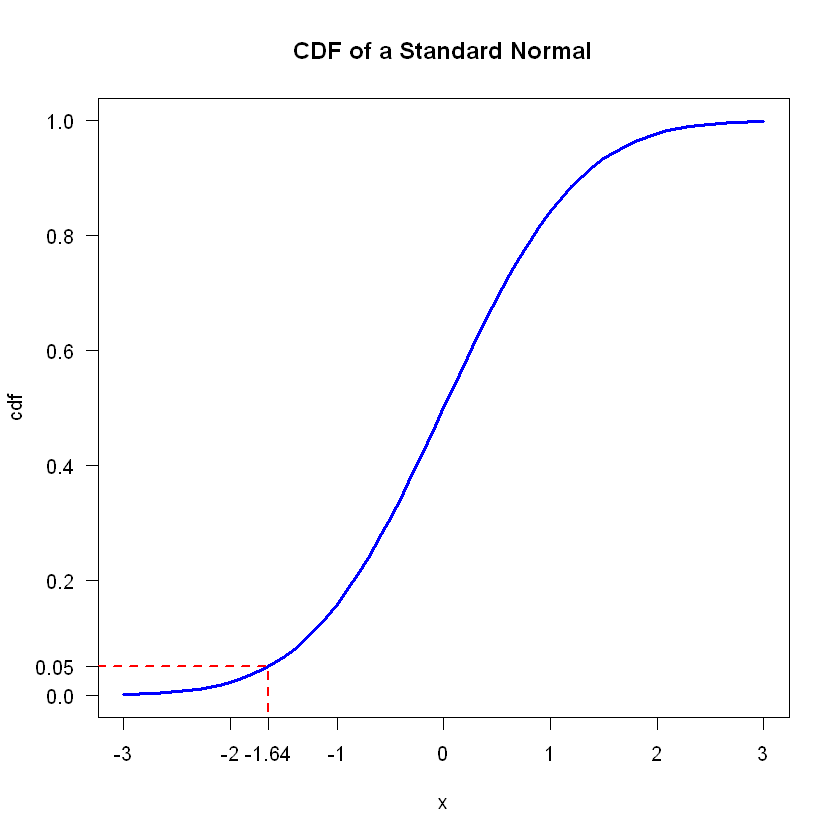### Comparing the Normal Distribution with the Student-t¶

The Student-t distribution has fatter tails than the Normal, which can be convenient for some applications. The lower the degrees of freedom of a Student-t, the fatter the tails. By definition, a Student-t will converge to a Normal distribution as the degrees of freedom go to infinity.

In :
# Start by creating a sequence
x <- seq(-3, 3, 0.1)

# Normal density
normal <- dnorm(x)

# Student-t with 2 df
st2 <- dt(x, df = 2)

# Student-t with 3 df
st3 <- dt(x, df = 3)

# Student-t with 10 df
st10 <- dt(x, df = 10)

plot(x, normal, type = "l", main = "Comparing distributions", col = 1, xlab = "x", ylab = "f(x)")
lines(x, st2, col = 2)
lines(x, st3, col = 3)
lines(x, st10, col = 4)

legend("topright", legend = c("Normal", "T - 2 df", "T - 3 df", "T - 10 df"), col = c(1:4), fill = c(1:4))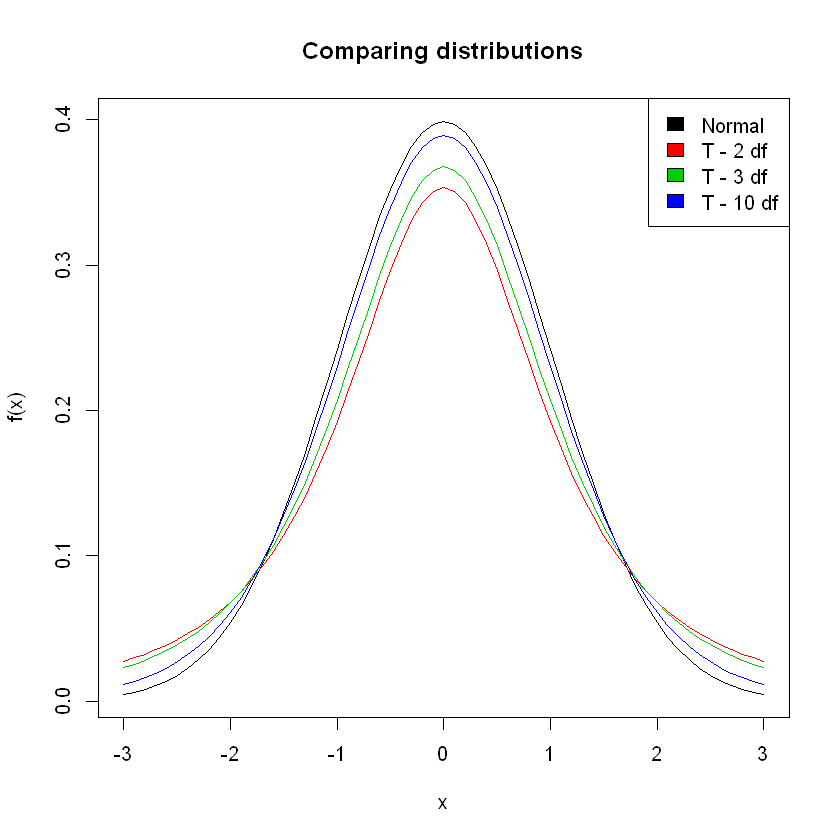## Random numbers¶

One of the advantages of working with statistical software is being able to do simulations using random (or better said, pseudorandom) numbers.

We can get random numbers drawn from a specific distribution by using the prefix r before the distribution name:

In :
# One random number from a Standard Normal
rnorm(1)

# Ten random numbers from a Normal(10, 0.5)
rnorm(10, mean = 10, sd = 0.5)

# One random number from a Student-t with 5 degrees of freedom
rt(1, df = 5)

0.745692509459398
1. 10.0060522617146
2. 10.2068990014442
3. 10.1242663460331
4. 10.1188317735893
5. 10.3810177201178
6. 9.32474277836731
7. 9.70614498971806
8. 9.67171136967637
9. 11.0695937836709
10. 9.99766338623383
-0.0923971115282568

Everytime we run a function that outputs random numbers, we will get a different value. Usually, to allow for replication, we set a seed:

In :
set.seed(442)
rnorm(5)

1. -0.894891360378309
2. -2.6921679536767
3. 0.764099073417597
4. -0.0172356169957856
5. -1.62406009659155
In :
set.seed(442)
rnorm(5)

1. -0.894891360378309
2. -2.6921679536767
3. 0.764099073417597
4. -0.0172356169957856
5. -1.62406009659155

### Comparing distributions using random numbers¶

To show the Fat Tails of the Student-t compared to the Normal distribution, we will draw 1000 points from each distribution:

In :
# Normal distribution
rnd_normal <- rnorm(1000)

# Student-t
rnd_t <- rt(1000, df = 3)

# Plotting
plot(rnd_t, col = "red", pch = 16, main = "Random points from a Normal and Student-t")
points(rnd_normal, col = "blue",pch = 16)

legend("bottomright", legend = c("Student-t", "Normal"), pch = 16, col = c("red", "blue"))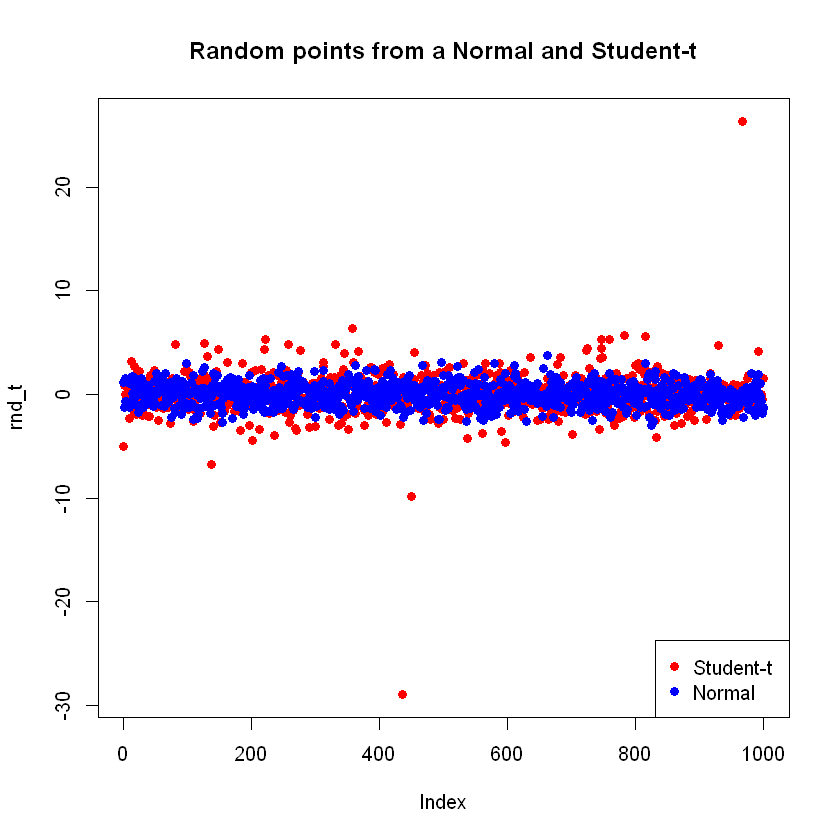We see that the points from a Student-t distribution take on more extreme values compared to the Normal. This is a consequence of fat tails.

Let's say I want to assign your grades for FM442 using a random number generator. Grades go from 0 to 100 and have to be integers. Drawing numbers from a distribution like the Normal or the Uniform will give us non-integers, so we can either:

• Round the number to the nearest integer
• Use a different function, like sample(), which only outputs integers

We will use the latter. sample(x,size) takes a vector x, and a size, and returns a vector of the given size of random draws from x:

In :
sample(1:100, 1)
sample(1:100, 3)
sample(1:100, 5)

44
1. 53
2. 10
3. 37
1. 9
2. 31
3. 20
4. 18
5. 86

Let's say there are 60 students in the class, we can get the grades:

In :
# Getting the grades

# Creating a histogram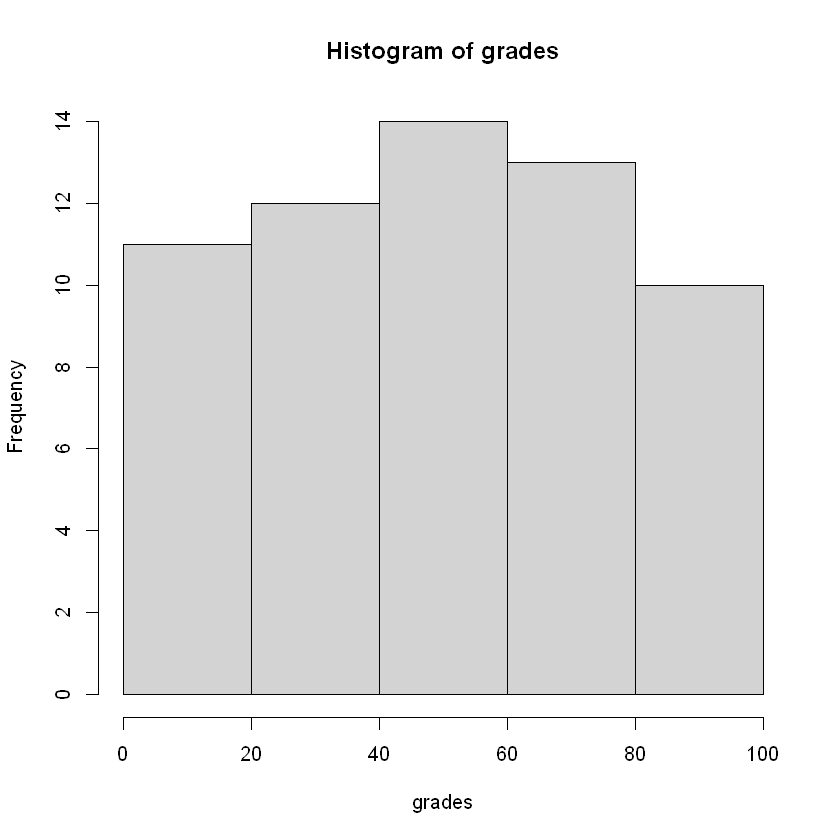How many people got a Distinction:

In :
# We can use a condition in the square brackets to subset the vector:

18

### Small and Large sample properties¶

Let's explore the distribution of random samples of different sizes, drawn from a standard normal distribution, compared to the distribution:

In :
# Sample size: 100
norm1 <- rnorm(100)

# Sample size: 1000
norm2 <- rnorm(1000)

# Sample size: 100000
norm3 <- rnorm(100000)

# Create a sequence
x <- seq(-3,3,0.1)

# Plot the histograms and overlay a normal distribution
hist(norm1, freq = FALSE, breaks = 20, main = "Sample size 100", col = "lightgrey", ylim = c(0, 0.5))
lines(x,dnorm(x), lwd = 3, col = "red")
hist(norm2, freq = FALSE, breaks = 20, main = "Sample size 1000", col = "lightgrey", ylim = c(0, 0.5))
lines(x,dnorm(x), lwd = 3, col = "red")
hist(norm3, freq = FALSE, breaks = 20, main = "Sample size 100000", col = "lightgrey", ylim = c(0, 0.5))
lines(x,dnorm(x), lwd = 3, col = "red")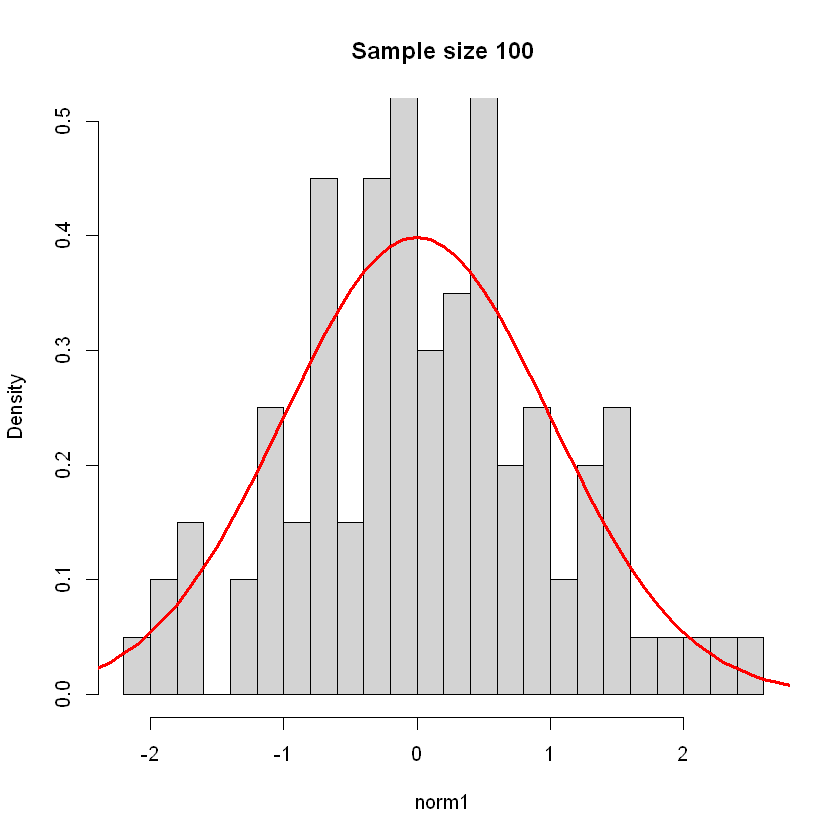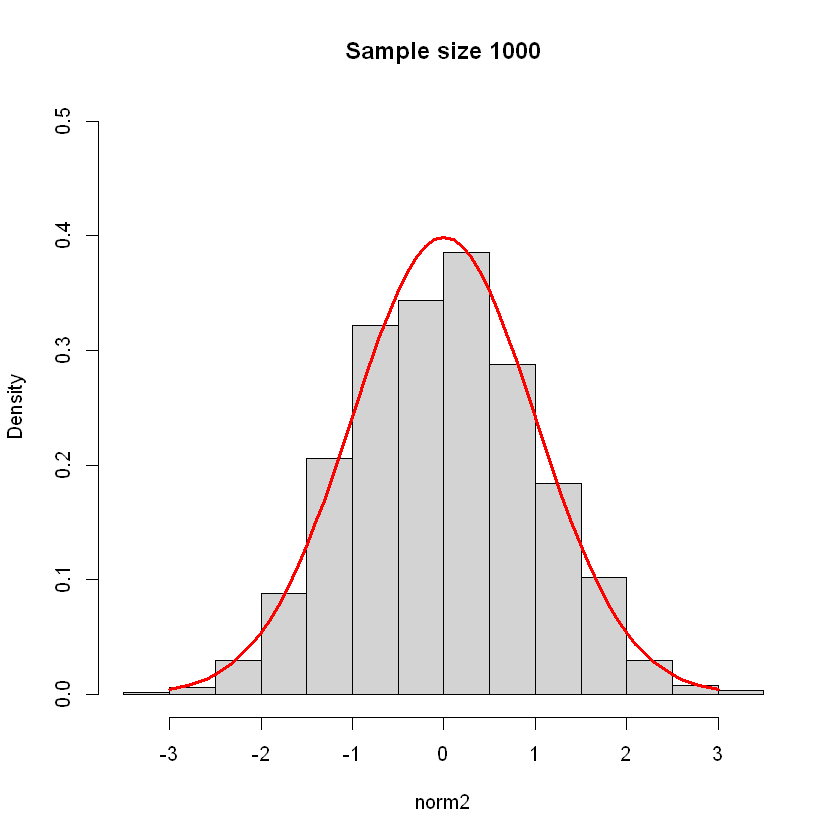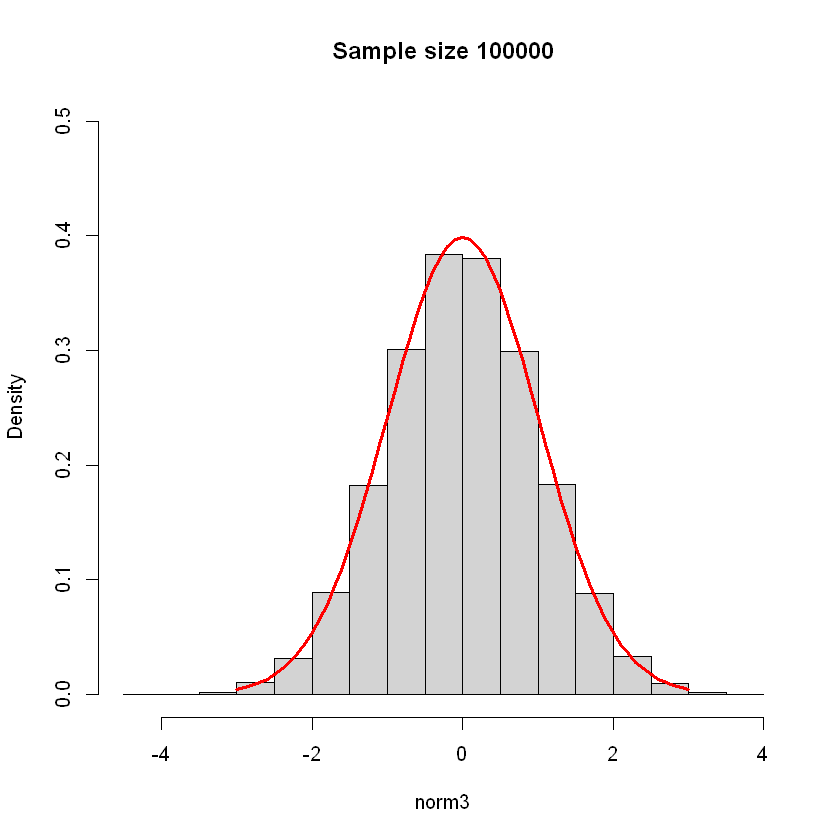## Visualizing and commenting on the price of a stock¶

As financial analysts, it is not enough to do pretty plots, simulations, and write a lot of code, we need to go beyond and try to tell a story and be able to understand and explain what has happened. In this section we will use the PRC.RData file we created to analyse the stock price of General Electric.

In :
load("PRC.RData")


And open the first rows to remember how it looks:

In :
head(PRC)

dateMSFTXOMGEJPMINTCCint_date
1990-01-020.6163194 12.5000 44.50000 10.00000 1.125000 22.87621 19900102
1990-01-030.6197917 12.3750 44.41667 10.33333 1.093750 23.16825 19900103
1990-01-040.6380208 12.2500 44.16667 10.37500 1.117188 22.87621 19900104
1990-01-050.6223958 12.1875 43.75000 10.41667 1.109375 23.07090 19900105
1990-01-080.6319444 12.3750 44.00000 10.41667 1.125000 23.26559 19900108
1990-01-090.6302083 12.1250 43.08333 10.08333 1.156250 22.97356 19900109

Now let's extract the information for General Electrics and plot the prices. The subset() function keeps the output as a data.frame. If we wanted the output as a vector, we could use PRC$GE: In : # Subset the PRC data frame GE <- subset(PRC, select = "GE") # Rename the column as price names(GE) <- "price" # Plot plot(GE$price, type = "l", main = "Price of GE")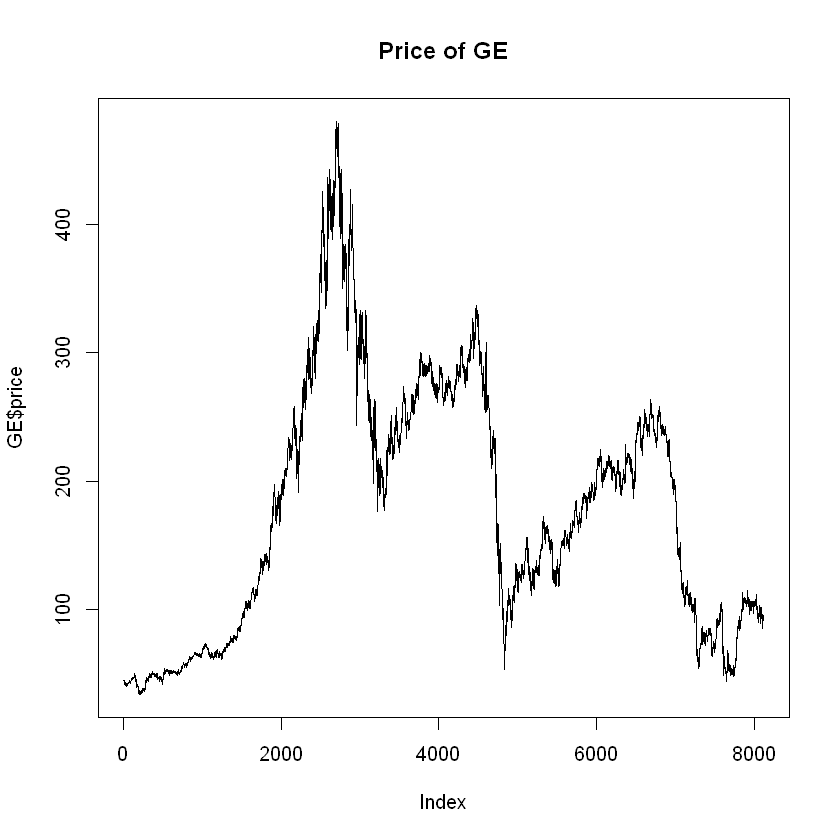This plot is useless for analysis without including dates:

In :
GE$date <- PRC$date
plot(GE$date, GE$price, type = "l", main = "Price of GE")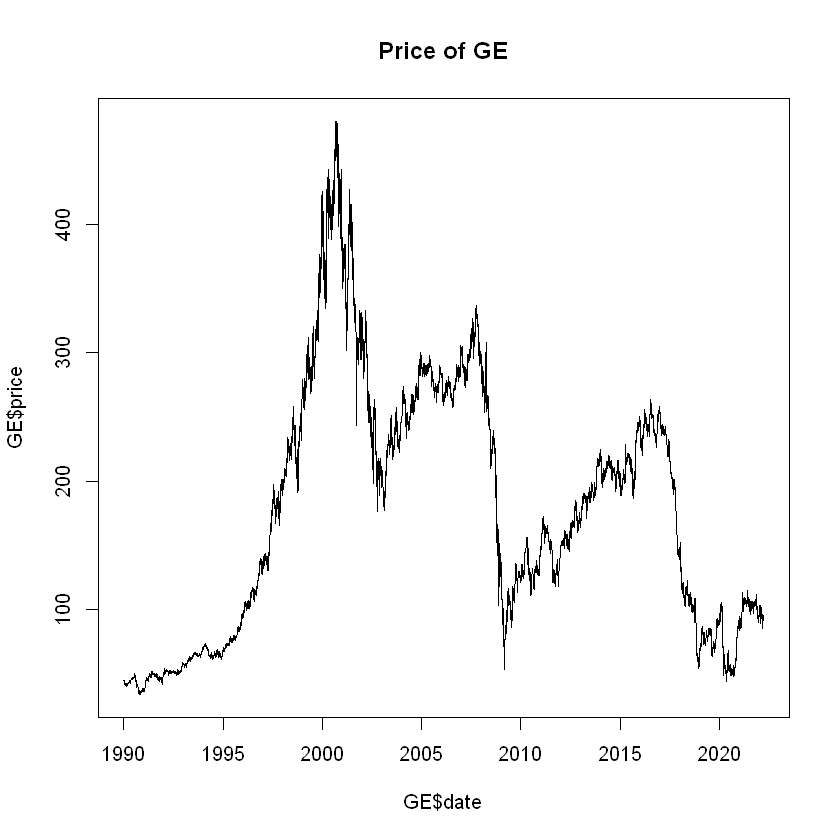We see General Electric doing well in the second half of the 90s, with an exponentially increasing price that reached a peak around 2000. The effect of the financial crisis is clear with a significant drop in the price around 2008.

First, let's find what the maximum price of GE was, and when it was reached:

In :
# Finding the highest price
max(GE$price) # We can find the date when this happened in two different but equivalent ways # 1. Filtering the dates when the stock price was at its maximum GE$date[GE$price == max(GE$price)]
# 2. Find the index where the maximum value is reached, and use it as a filter
GE[which.max(GE$price),]  480 pricedate 2694480 2000-08-28 Jack Welch, who was the CEO of GE for 20 years, retired on September 7, 2001. He was considered to be one of the most valuable CEOs of all time. Let's add a vertical line on our plot to reflect this: In : # First we plot the data plot(GE$date, GE$price, type = "l", main = "Price of GE") # We add a vertical line using abline and the function ymd to turn a number into a Date abline(v = ymd(20010907), lwd = 2, col = "blue")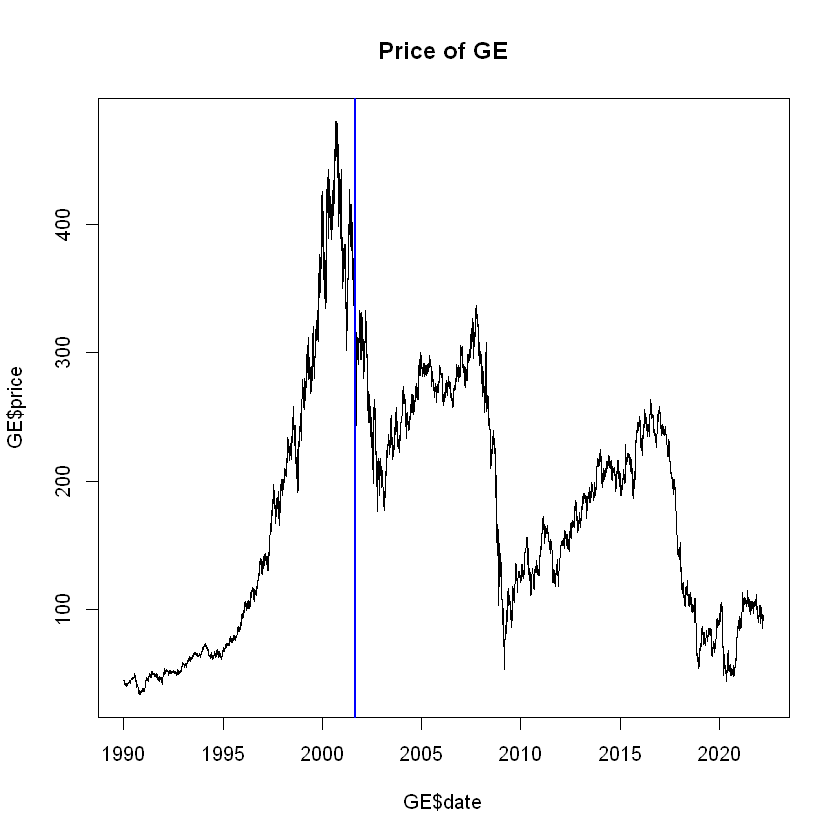Now let's zoom into the crisis in 2008, using the xlim option: In : # First we create the limits x_left = ymd(20080101) x_right = ymd(20120101) # Use xlim to incorporate them plot(GE$date, GE$price, type = "l", main = "Price of GE during the crisis", xlab = "Time", ylab = "Price", xlim = c(x_left, x_right))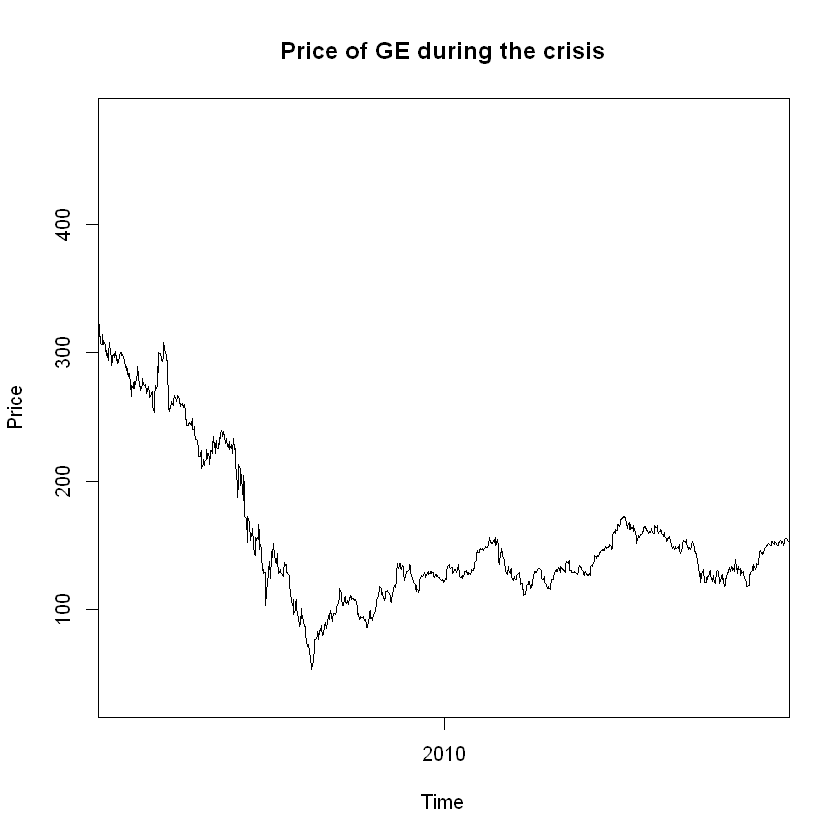We now have a plot that indeed zooms into the price of GE during the crisis, which is what we wanted. However, the plot is again useless if we do not format the x-axis to see the dates. We use axis.Date() to edit the x-axis. The first argument side decides which axis will be changed. The input of argument at is date type object. In : # Create the plot again plot(GE$date, GE$price, type = "l", main = "Price of GE during the crisis", xlab = "Time", ylab = "Price", xlim = c(x_left, x_right), xaxt = "na") # Use axis.Date to edit axis.Date(side = 1, at=seq(x_left, x_right, by="6 mon"), format="%m/%Y")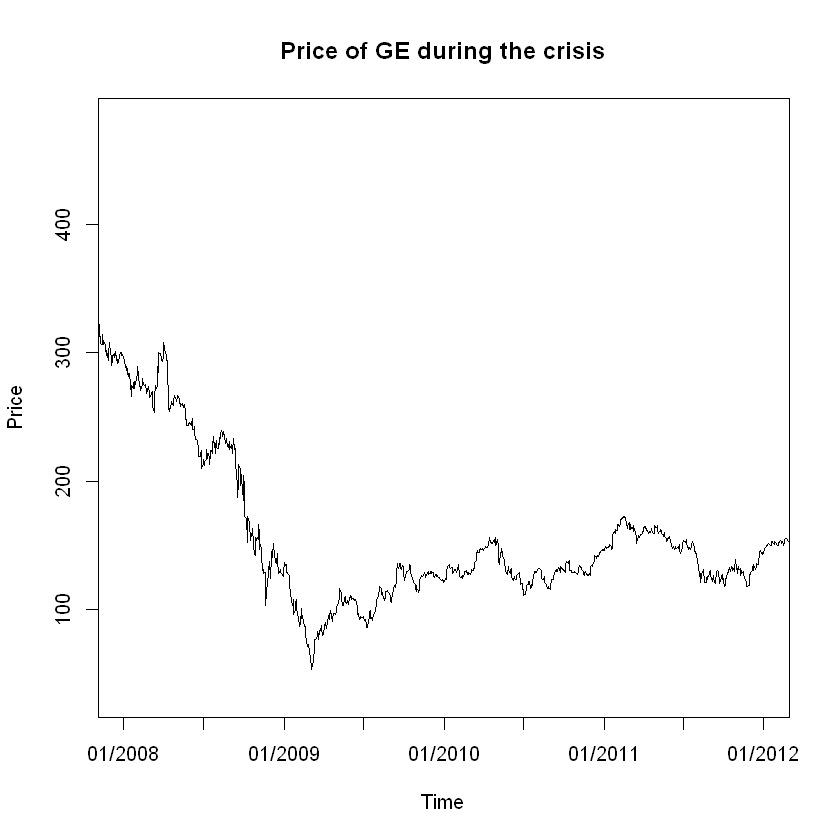It is clear that GE, as many other firms, suffered during the financial crisis. But we want to tell a story that goes beyond just presenting a plot. Every piece of financial data that we use needs to be accompanied by research. In the particular case of General Electric, one of the main reasons of its profitable situation until early 2008 was GE Capital, a subsidiary of the firm that focused on financial services. GE Capital almost went bust in the crisis due to its high leverage, which had a significant effect on its parent company. To continue our analysis, we will load the RET.RData data frame and add a column to GE with the firm's returns: In : # Loading the data load("Y.RData") # Adding the returns to our GE data frame GE$returns <- Y$GE # Plot the returns plot(GE$date, GE$returns, type = "l", main = "Returns of GE")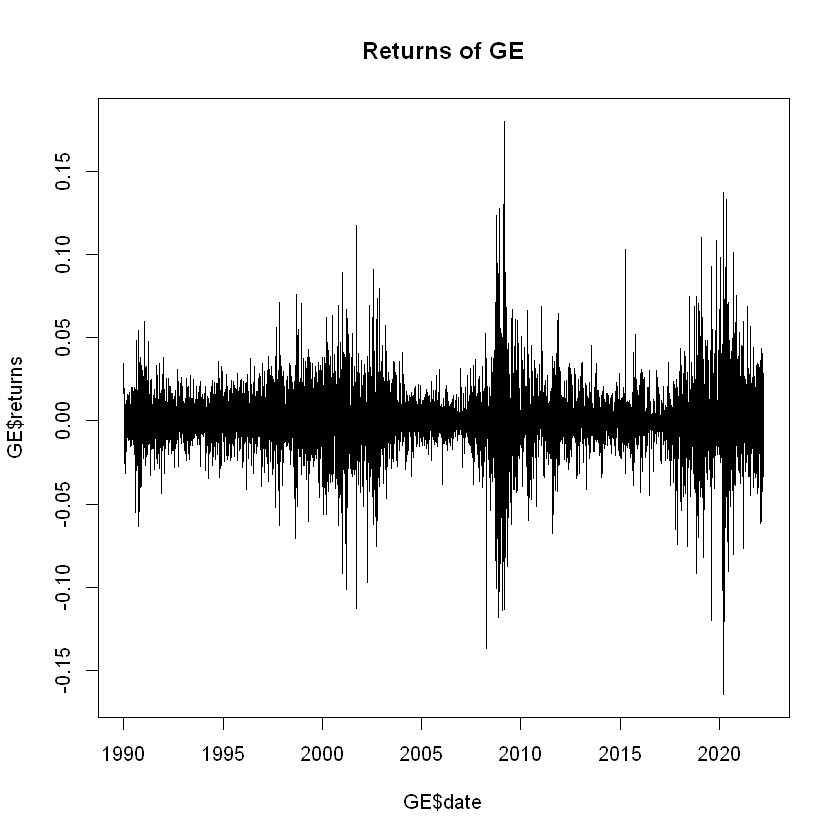This plot shows clearly shows the volatility clusters discussed in class. As we expect, there is a large volality cluster during the crisis. There is another more recent cluster. On October 1st, 2018, the company decided to fire its CEO unexpectedly. Let's zoom into this period to see if there was an effect: In : x_left = ymd(20170101) x_right = ymd(20191231) plot(GE$date, GE$returns, type = "l", main = "Returns of GE between 2017 and 2019", xlab = "Time", ylab = "Returns", xlim = c(x_left, x_right), xaxt = "na") axis.Date(1, at=seq(x_left, x_right, by="1 mon"), format="%m/%Y")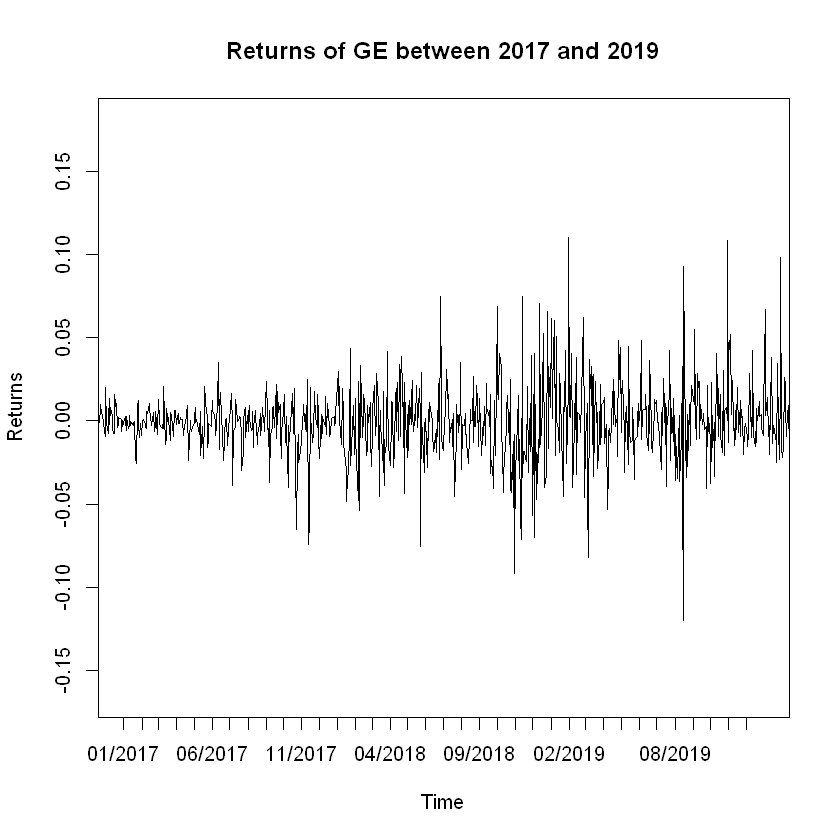Whenever we want to talk about a stock, part of the story is external, like the economy, but another part of it is internal, like firing the CEO. It is important to always take both into account when performing an analysis. ## Graphical Analysis and Statistical Tests¶ ### Normality of returns¶ Returns are not normally distributed. Financial data exhibits fat tails, which means that extreme values, both positive and negative, are seen more frequently than what we would expect if the data followed a normal distribution. Also, in financial data most days are uneventful, so we see a higher frequency of points around zero than in a normal distribution. To show this graphically, we will plot the histogram of returns for GE and overlay a normal distribution with the same mean and standard deviation: In : # Get the mean and sd of returns ge_mean <- mean(GE$returns)
ge_sd <- sd(GE$returns) paste0("Mean: ", round(ge_mean,3)) paste0("Standard Deviation: ", round(ge_sd,3)) # Create the histogram hist(GE$returns, freq = FALSE, main = "Returns of GE", col = "lightgrey", breaks = 50)

x <- seq(-3,3,0.001)
lines(x, dnorm(x, mean = ge_mean, sd = ge_sd), lwd = 3, col = "red")

'Mean: 0'
'Standard Deviation: 0.019'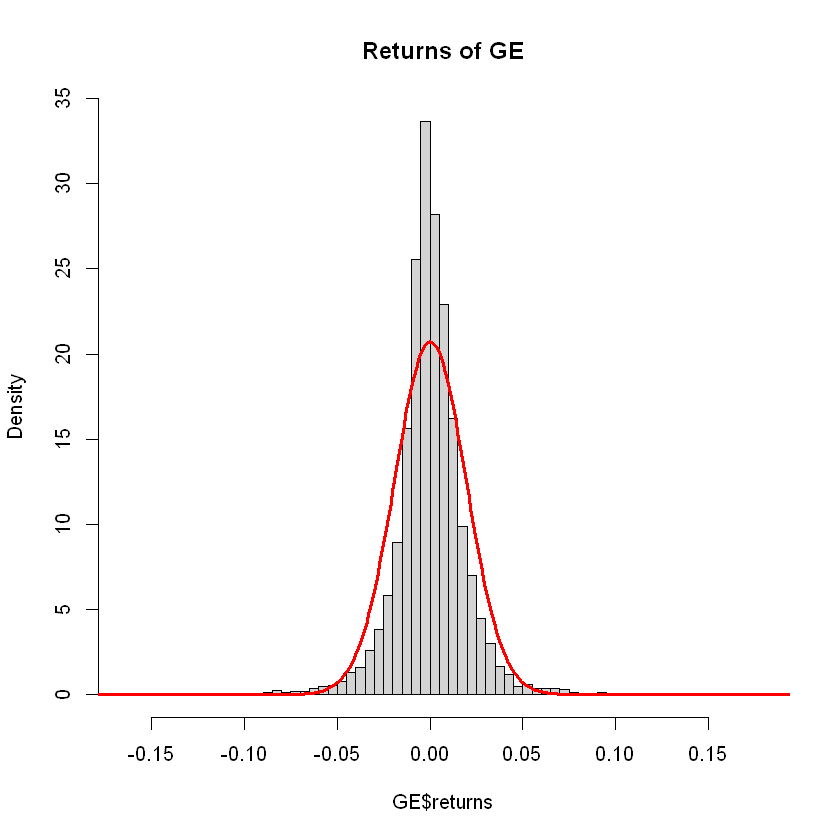It is visually obvious that the returns do not follow a normal distribution with matched moments. However, visually obvious is not a rigurous statement, so we should perform a statistical test to prove this.

To see if a vector of numbers follows could have been drawn from a normal distribution, we will use the Jarque-Bera test, which uses skewness and kurtosis. The test statistic of the Jarque-Bera test asymptotically follows a chi-square distribution with two degrees of freedom. The null hypothesis, $H_0$, of the test is that the skewness and excess kurtosis of a distribution are jointly zero, which is the equivalent of a Normal Distribution. This test can be directly implemented with the jarque.bera.test() function from the tseries package:

In :
jarque.bera.test(GE$returns)   Jarque Bera Test data: GE$returns
X-squared = 22053, df = 2, p-value < 2.2e-16


To understand the output of a test, we can look at the p-value. A p-value below 0.05 tells us that we have enough evidence to reject the null hypothesis $H_0$ with a confidence level of 95%. Statistically, the p-value is the inverse of the test-statistic under the CDF of the asymptotic distribution.

The p-value of this test is basically zero, which means that we have enough evidence to reject the null hypothesis that our data has been drawn from a Normal distribution.

### Fat tails and QQ-Plots¶

Other distributions that exhibit fat tails might be more suitable to work with. A common choice is the Student-t distribution. A graphical way to see how our data fits different distributions is by using a Quantile-Quantile Plot. This method plots the quantiles of our data against the quantiles of a specified distribution. If the distribution is a good fit for our data, we will see the data points aligning with the diagonal line.

To build QQ plots, we will use the qqPlot function from the car package:

In :
# qqPlot of the normal distribution
qqPlot(GE$returns, distribution = "norm", envelope = FALSE)  1. 4837 2. 7610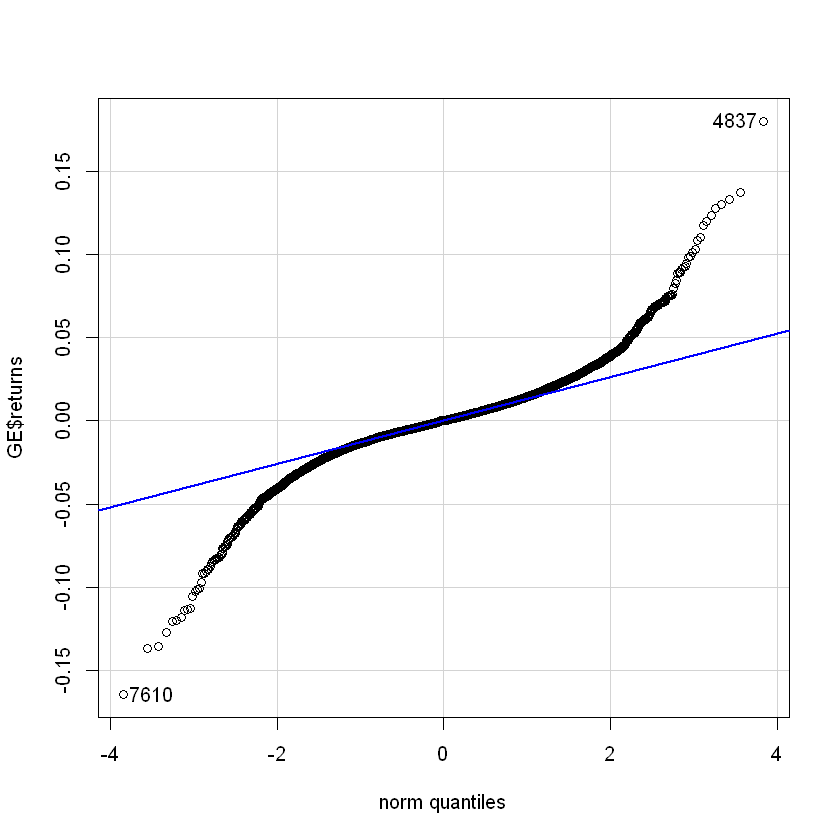We expect our data to have a better fit with a Student-t. To find out the degrees of freedom to use, we will do different qqPlots: In : # 2 degrees of freedom qqPlot(GE$returns, distribution = "t", df = 2, envelope = FALSE,
main = "2 Degrees of Freedom")

# 3 degrees of freedom
qqPlot(GE$returns, distribution = "t", df = 3, envelope = FALSE, main = "3 Degrees of Freedom") # 4 degrees of freedom qqPlot(GE$returns, distribution = "t", df = 4, envelope = FALSE,
main = "4 Degrees of Freedom")

1. 4837
2. 7610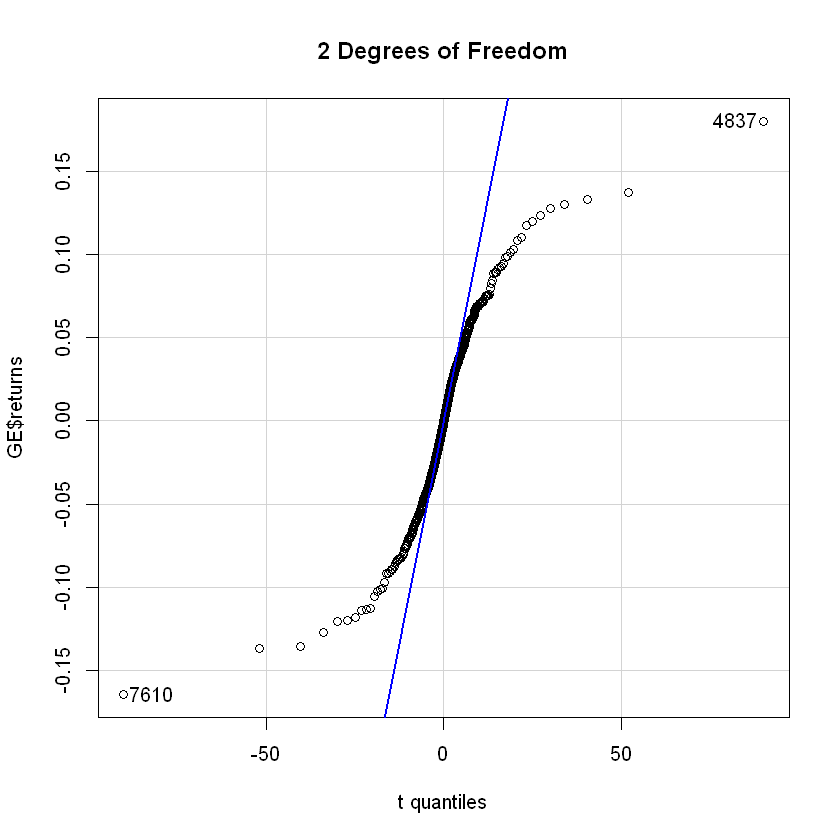1. 4837
2. 7610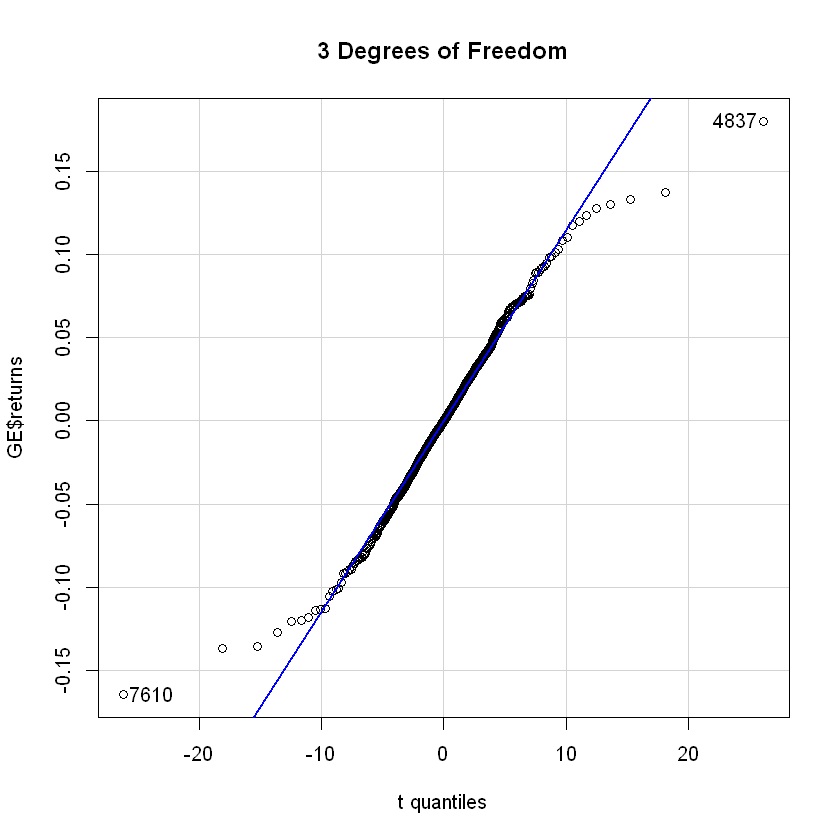1. 4837
2. 7610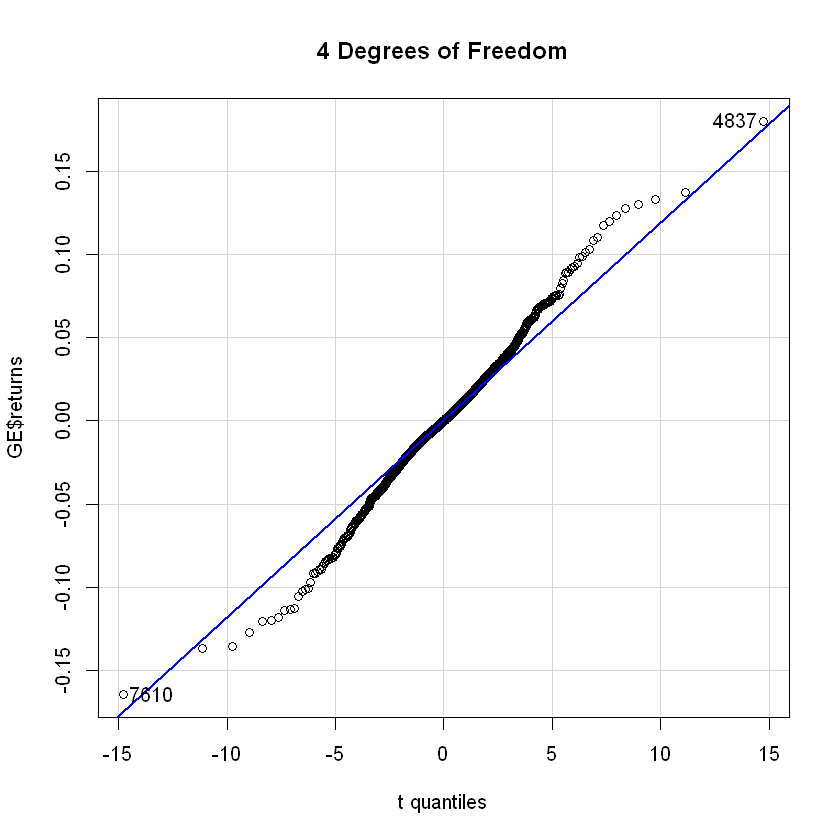The best fit seems to be a Student-t with 3 degrees of freedom.

### Autocorrelation¶

The autocorrelation function shows us the linear correlation between a value in our time series with its different lags. For example, if tomorrow's return can be determined with today's value, we would expect to have a significant autocorrelation of lag one.

The acf function plots the autocorrelation of an ordered vector. The horizontal lines are confidence intervals, meaning that if a value if outside of the interval, it is considered significantly different from zero.

Let's first take a look at the acf of returns:

In :
# Autocorrelation of returns
acf(GE$returns, main = "Autocorrelation of returns")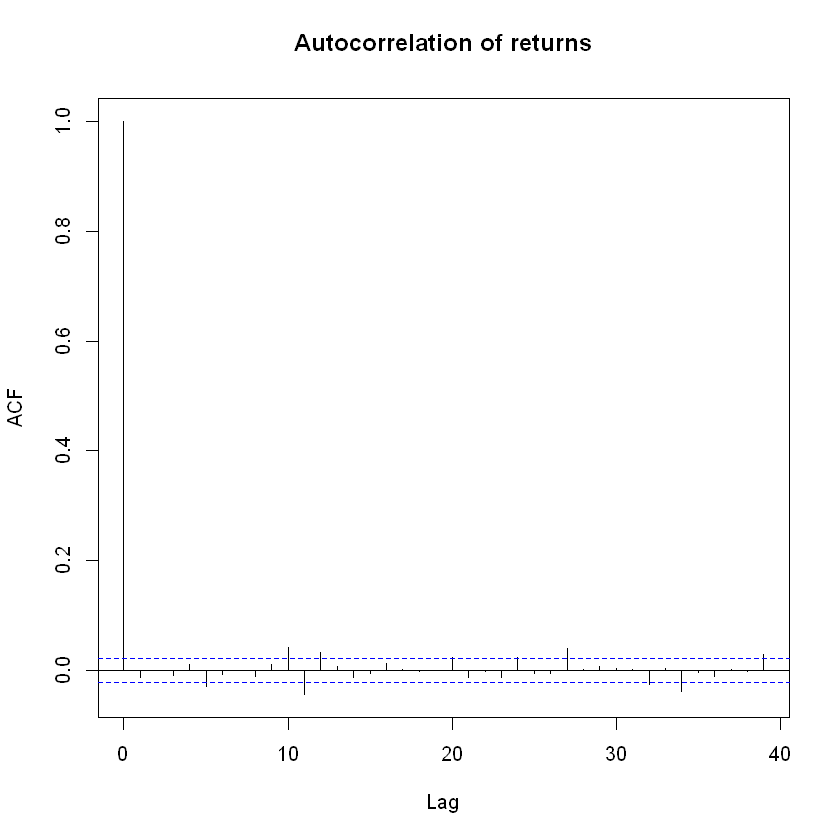The autocorrelation of lag 0 is always 1 (every value is perfectly correlated with itself), but apart from that, we see no significant values. Remember than with a 95% confidence interval, we would expect to see 1 out of every 20 values to show significance out of pure chance. This shows us good evidence that you cannot easily forecast stock prices. If there was a clear autocorrelation, you could build trading strategies to create profit, but the market makes sure this is not the case. Even if returns show no autocorrelation, let's see what happens with returns squared, which are our estimate for volatility: In : # Autocorrelation of returns squared acf(GE$returns^2, main = "Autocorrelation of returns squared")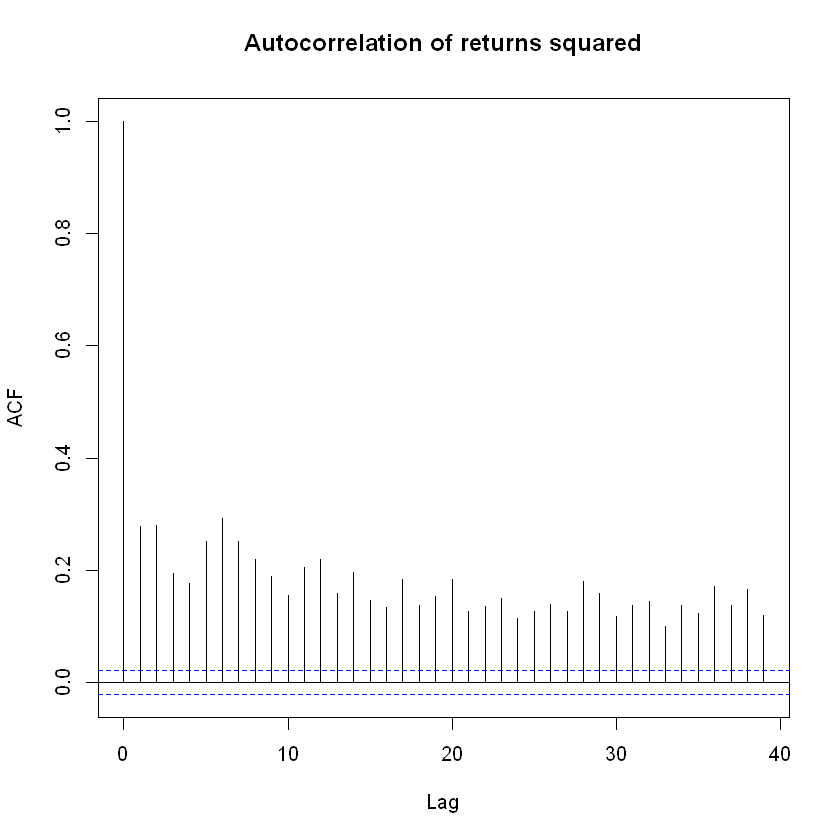We clearly see there is a strong positive autocorrelation for all shown lags. This pattern is normally seen in long memory time series. Recall that in a GARCH model, the size of $\alpha + \beta$ determines the memory of the time series. We will discuss more of the GARCH properties in lecture. We can extend the lags up to 100:

In :
# Autocorrelation of returns squared, 100 lags
acf(GE$returns^2, main = "Autocorrelation of returns squared - 100 lags", lag.max = 100)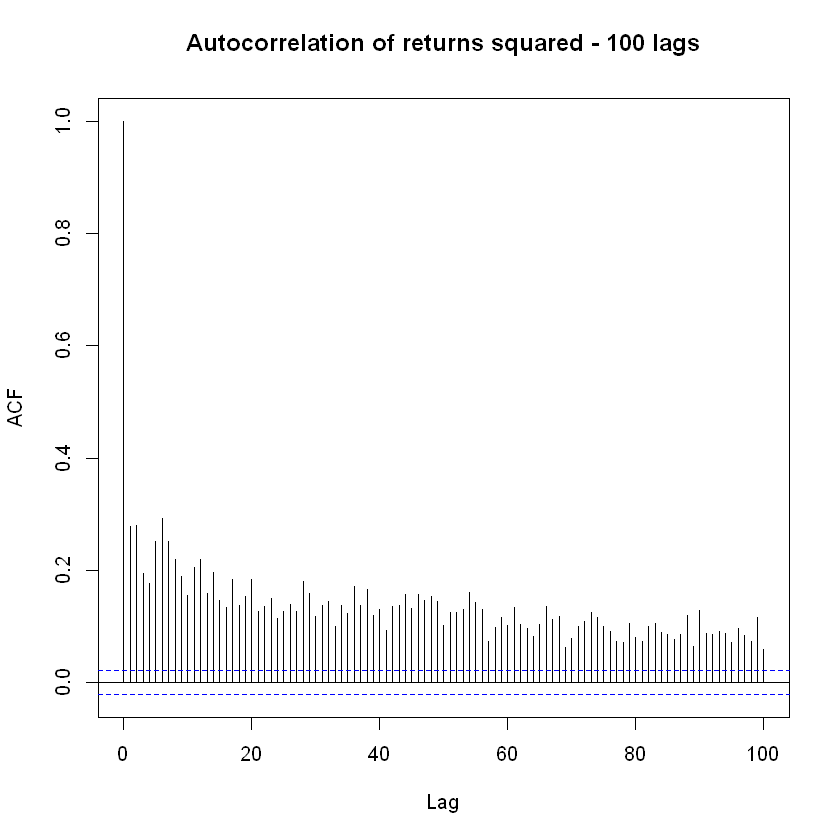fpp2 library's ggAcf function can also provide the acf plot with ggplot features. ggAcf could be a better looking alternative. We can use the Ljung-Box test to test for serial correlation. This is a statistical tesf of whether any autocorrelations of a time series are different from zero. The null hypothesis$H_0$is that the data are independently distributed, while$H_1$is that the data exhibit serial correlation. The test statistic is distributed as a chi-square. The function Box.test can perform this test: In : Box.test(GE$returns, type = "Ljung-Box")
Box.test(GE$returns^2, type = "Ljung-Box")   Box-Ljung test data: GE$returns
X-squared = 1.366, df = 1, p-value = 0.2425

	Box-Ljung test

data:  GE\$returns^2
X-squared = 625.14, df = 1, p-value < 2.2e-16


## Recap¶

In this seminar we have covered:

• Using R to work with CDF, inverse CDF, and PDF of various statistical distributions
• Drawing random numbers from specified distributions
• Using seeds for replicability
• Small and large sample properties of random numbers drawn from a distribution
• Analyzing and commenting on the price of a stock
• Telling a financial story using data as support
• Testing for normality
• Creating QQ-plots
• Autocorrelation function
• Importing a function

Some new functions used:

• pnorm()
• dnorm()
• qnorm()
• rnorm()
• set.seed()
• quantile()
• abline()
• axis.Date()
• jarque.bera.test()
• qqPlot()
• acf()
• source()`

For more discussion on the material covered in this seminar, refer to Chapter 1: Financial markets, prices and risk on Financial Risk Forecasting by Jon Danielsson.

Acknowledgements: Thanks to Alvaro Aguirre and Yuyang Lin for creating these notebooks